Möbius band

immersion via f: the domain of f:D→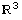is a subset D⊂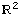around 0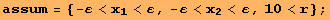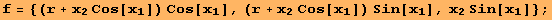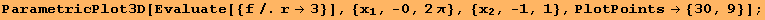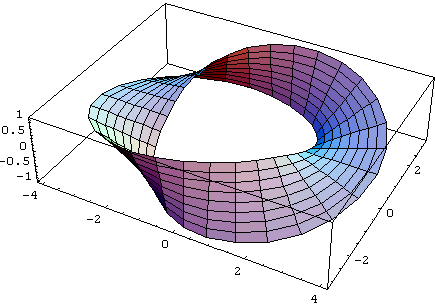The metric tensor g on D⊂is the pullback of the standard metric tensor Id onvia f.The following expression is the (euclidean) length of "going once around". The length is minimal "walking in the middle", i.e. when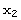=0. In this case, the length is simply 2, the circumferrence of a circle.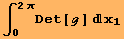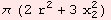Have a look at the standard tensors of the sphere: g, Γ, R, Ric, S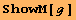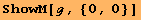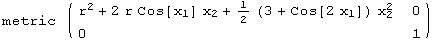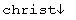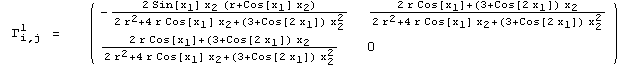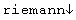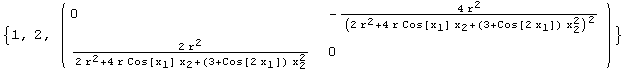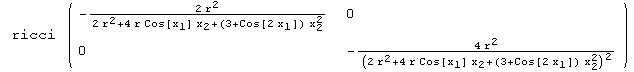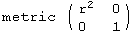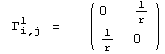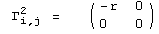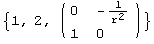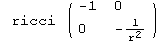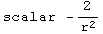For some reason the following line takes forever to execute. In the next line we introduce a new coordinate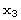, which parametrizes the local normal vector field.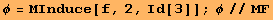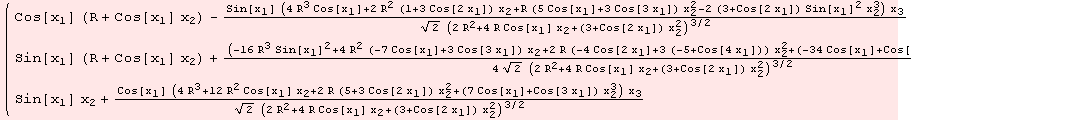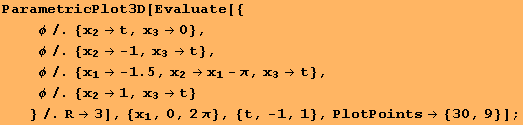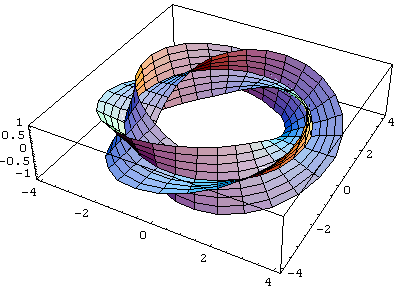Created by Mathematica  (December 22, 2006)